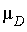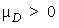Dependent Means

There are two possible cases when testing two population means, the dependent case and the independent case. Most books treat the independent case first, but I'm putting the dependent case first because it follows immediately from the test for a single population mean in the previous chapter.

The Mean of the Difference:The idea with the dependent case is to create a new variable, D, which is the difference between the paired values. You will then be testing the mean of this new variable.

4. Convert to proper notation: